# EVA财务指标，企业eva增长率预测

EVA =税后净营业利润-资本成本

=税后净营业利润-资本总额*WACC

EVA率=税后净营业利润/资本总额-WACC

=税后净营业利润/营业收入*营业收入/资本总额-WACC

=税后净营业利润率*资本周转率-WACC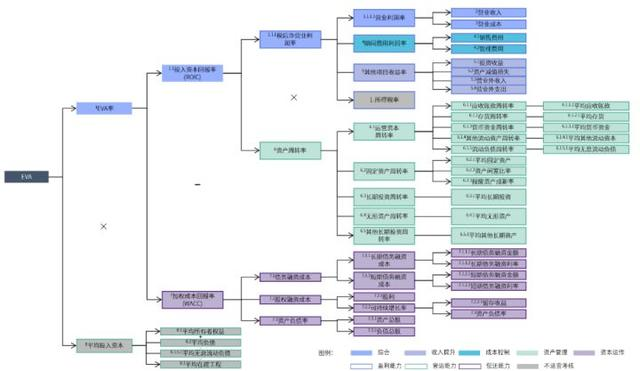EVA指标分解体系的意义在于，当EVA变化时能够通过指标的层层分解，找到EVA变化的原因。

1

``````# EVA =税后净营业利润-平均资本成本=税后净营业利润-资本总额*WACC
EVA = pd.DataFrame()
EVA['净营业利润'] = p_l.loc['五、净利润']
EVA['股东权益'] = bal_sht.loc['所有者权益(或股东权益)合计']
EVA['有息负债'] = bal_sht.loc['短期借款']+bal_sht.loc['长期借款']+bal_sht.loc['应付债券']
EVA['资本总额'] = EVA['股东权益']+EVA['有息负债']-bal_sht.loc['在建工程']
#我们已经学过WACC的计算公式WACC=(K_e×W_e )+(K_d [1-T]×W_d)

#由于权益和负债的资本成本较难获得，这里我们用
#权益净利率代替权益资本成本
#用利息现金流/有息负债代替债务资本成本
#用负债和权益的占比代表权重

EVA['Ke']=KPI['权益资本利润率']
EVA['Kd']=cash_flow.loc['分配股利、利润或偿付利息所支付的现金']/(EVA['有息负债']+EVA['有息负债'].shift(-1))*2
EVA['WACC']=EVA['Ke']*EVA['股东权益']/
(EVA['股东权益'] +EVA['有息负债'])\
+EVA['Kd']*(EVA['有息负债'])/
(EVA['股东权益']+EVA['有息负债'])*(1-0.25)
EVA['EVA'] = EVA['净营业利润']-EVA['资本总额']*EVA['WACC']
EVA
EVA['EVA'] = EVA['净营业利润']-EVA['资本总额']*EVA['WACC']
EVA
``````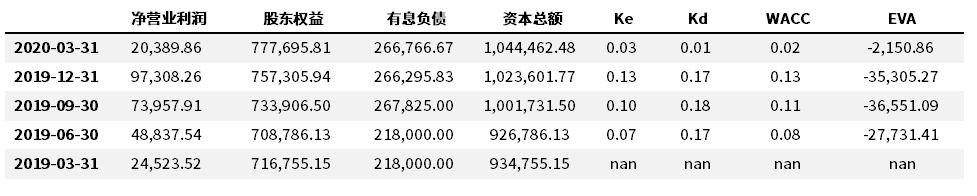2

``````  EVA-EVA.shift(-1)
``````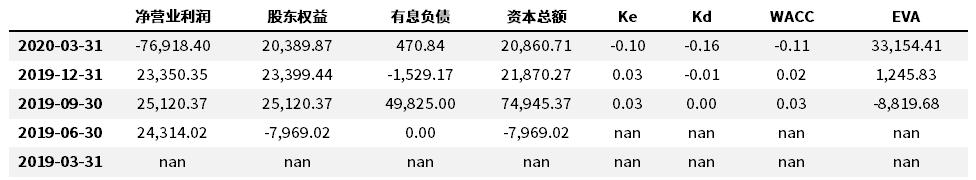3

``````# EVA率=税后净营业利润率*资本周转率-WACC
EVA['税后净营业利润率']=KPI['销售利润率']
EVA['资本周转率']=(p_l.loc['一、营业总收入']/EVA['资本总额'])
EVA['EVA率']=EVA['税后净营业利润率']*EVA['资本周转率']-EVA['WACC']
#EVA['EVA率2']=EVA['EVA']/EVA['资本总额'] ---两种计算结果是一致的
EVA
``````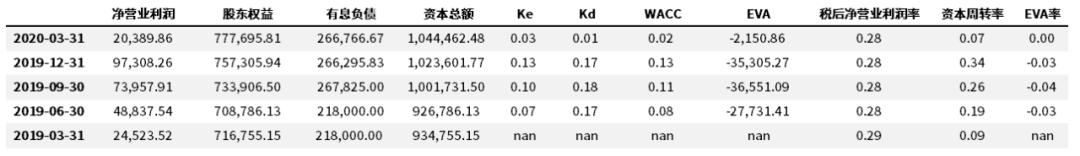``````EVA_P=EVA[['税后净营业利润率‘,
’资本周转率','WACC','EVA率']]*100
EVA_P-EVA_P.shift(-1)
``````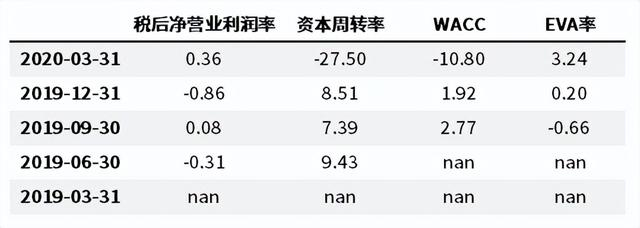?图片版权归财码数字化所有，如对版权有异议，请联系后台议定处理。